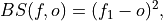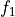# climpred.metrics._brier_score#

climpred.metrics._brier_score(forecast: xarray.Dataset, verif: xarray.Dataset, dim: Optional[Union[str, List[str]]] = None, **metric_kwargs: Any) [source]#

Brier Score for binary events.

The Mean Square Error (`mse`) of probabilistic two-category forecasts where the verification data are either 0 (no occurrence) or 1 (occurrence) and forecast probability may be arbitrarily distributed between occurrence and non-occurrence. The Brier Score equals zero for perfect (single-valued) forecasts and one for forecasts that are always incorrect.whereis the forecast probability of.

Note

The Brier Score requires that the observation is binary, i.e., can be described as one (a “hit”) or zero (a “miss”). So either provide a function with with binary outcomes `logical` in `metric_kwargs` or create binary verifs and probability forecasts by hindcast.map(logical).mean(“member”). This Brier Score is not the original formula given in Brier .

Parameters
• forecast – Raw forecasts with `member` dimension if `logical` provided in metric_kwargs. Probability forecasts in `[0, 1]` if `logical` is not provided.

• verif – Verification data without `member` dim. Raw verification if `logical` provided, else binary verification.

• dim – Dimensions to aggregate. Requires `member` if `logical` provided in `metric_kwargs``to create probability forecasts. If ``logical` not provided in `metric_kwargs`, should not include `member`.

• logical (callable) – Function with bool result to be applied to verification data and forecasts and then `mean("member")` to get forecasts and verification data in interval `[0, 1]`. see `xskillscore.brier_score()`

Notes

 minimum 0.0 maximum 1.0 perfect 0.0 orientation negative

References

Example

Define a boolean/logical: Function for binary scoring:

```>>> def pos(x):
...     return x > 0  # checking binary outcomes
...
```

Option 1. Pass with keyword `logical`: (specifically designed for `PerfectModelEnsemble`, where binary verification can only be created after comparison)

```>>> HindcastEnsemble.verify(
...     metric="brier_score",
...     comparison="m2o",
...     dim=["member", "init"],
...     alignment="same_verifs",
...     logical=pos,
... )
<xarray.Dataset>
Coordinates:
* lead     (lead) int32 1 2 3 4 5 6 7 8 9 10
skill    <U11 'initialized'
Data variables:
SST      (lead) float64 0.115 0.1121 0.1363 0.125 ... 0.1654 0.1675 0.1873
Attributes:
skill_calculated_by_function:  HindcastEnsemble.verify()
number_of_initializations:     64
number_of_members:             10
alignment:                     same_verifs
metric:                        brier_score
comparison:                    m2o
dim:                           ['member', 'init']
reference:                     []
logical:                       Callable
```

Option 2. Pre-process to generate a binary multi-member forecast and binary verification product:

```>>> HindcastEnsemble.map(pos).verify(
...     metric="brier_score",
...     comparison="m2o",
...     dim=["member", "init"],
...     alignment="same_verifs",
... )
<xarray.Dataset>
Coordinates:
* lead     (lead) int32 1 2 3 4 5 6 7 8 9 10
skill    <U11 'initialized'
Data variables:
SST      (lead) float64 0.115 0.1121 0.1363 0.125 ... 0.1654 0.1675 0.1873
Attributes:
skill_calculated_by_function:  HindcastEnsemble.verify()
number_of_initializations:     64
number_of_members:             10
alignment:                     same_verifs
metric:                        brier_score
comparison:                    m2o
dim:                           ['member', 'init']
reference:                     []
```

Option 3. Pre-process to generate a probability forecast and binary verification product. because `member` not present in `hindcast` anymore, use `comparison="e2o"` and `dim="init"`:

```>>> HindcastEnsemble.map(pos).mean("member").verify(
...     metric="brier_score",
...     comparison="e2o",
...     dim="init",
...     alignment="same_verifs",
... )
<xarray.Dataset>
Coordinates:
* lead     (lead) int32 1 2 3 4 5 6 7 8 9 10
skill    <U11 'initialized'
Data variables:
SST      (lead) float64 0.115 0.1121 0.1363 0.125 ... 0.1654 0.1675 0.1873
Attributes: